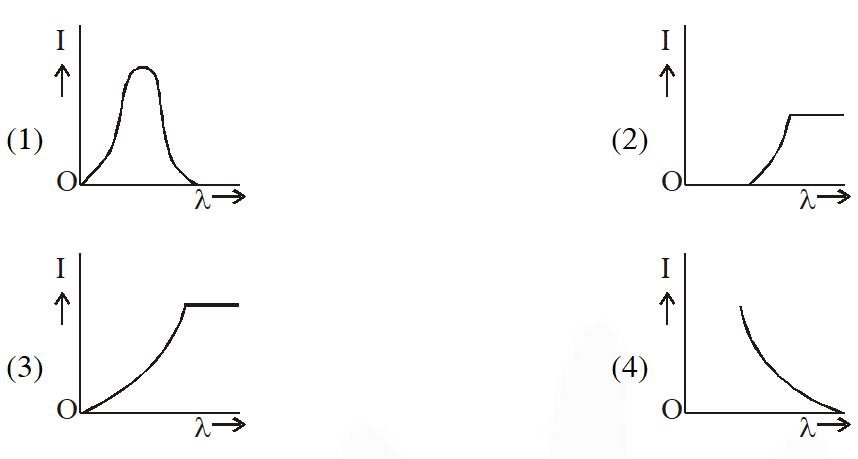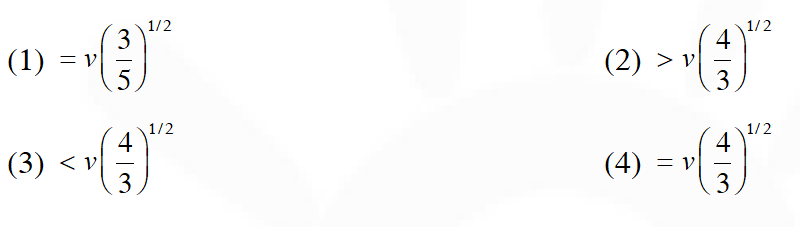Most Affordable JEE | NEET | 8,9,10 Preparation by Kota's Top IITian Doctor Faculties

# Photoelectric Effect - JEE Main Previous Year Questions with SolutionsJEE Main Previous Year Question of Physics with Solutions are available here. Practicing JEE Main Previous Year Papers Questions of Physics will help all the JEE aspirants in realizing the question pattern as well as help in analyzing their weak & strong areas. Get detailed Class 11th &12th Physics Notes to prepare for Boards as well as competitive exams like IIT JEE, NEET etc. eSaral helps the students in clearing and understanding each topic in a better way. eSaral is providing complete chapter-wise notes of Class 11th and 12th both for all subjects. Besides this, eSaral also offers NCERT Solutions, Previous year questions for JEE Main and Advance, Practice questions, Test Series for JEE Main, JEE Advanced and NEET, Important questions of Physics, Chemistry, Math, and Biology and many more. Download eSaral app for free study material and video tutorials.   Simulator   Previous Years AIEEE/JEE Mains Questions
Q. The surface of a metal is illuminated with the light of 400 nm. The kinetic energy of the ejected photoelectrons was found to be 1.68 eV. The work function of the metal is : (hc = 1240 eV-nm) (1) 1.51 eV            (2) 1.68 eV          (3) 3.09 eV              (4) 1.41 eV [AIEEE - 2009]
Ans. (4) $\mathrm{E}_{\mathrm{k}}=\frac{\mathrm{hc}}{\lambda}-\phi_{0} \Rightarrow 1.68=\frac{12400}{4000}-\phi_{0}$ By solving it $\phi_{0}=1.42 \mathrm{eV}$
Q. Statement-1 : When ultraviolet light is incident on a photocell, its stopping potential is V0 and the maximum kinetic energy of the photoelectrons is $\mathrm{K}_{\mathrm{max}}$. When the ultraviolet light is replaced by X-rays, both $\mathrm{V}_{0}$ and $\mathrm{K}_{\mathrm{max}}$ increase. Statement-2 : Photoelectrons are emitted with speeds ranging from zero to a maximum value because of the range of frequencies present in the incident light (1) Statement–1 is true, Statement–2 is false (2) Statement–1 is true, Statement–2 is true; Statement–2 is the correct explanation of Statement– 1 (3) Statement–1 is true, Statement–2 is true; Statement–2 is not the correct explanation of Statement– 1 (4) Statement–1 is false, Statement–2 is true [AIEEE - 2010]
Ans. (1) Speed of emitted electrons is independent of frequency of incident light.
Q. This question has Statememtn-1 and Statement-2. Of the four choices given after the statements, choose the one that best describes the two statements. Statement–1 : A metallic surface is irradiated by a monochromatic light of frequency $\mathrm{v}>\mathrm{v}_{0}$ (the threshold frequency). The maximum kinetic energy and the stopping potential are $\mathrm{K}_{\max }$ and $\mathrm{V}_{0}$ respectively. If the frequency incident on the surface is doubled, both the $\mathrm{K}_{\max }$ and $\mathrm{V}_{0}$ are also boubled. Statement-2 : The maximum kinetic energy and the stopping potential of photoelectrons emitted from a surface are linearly dependent on the frequency of incident light. (1) Statement–1 is true, Statement–2 is true, Statement–2 is not the correct explanationof Statement– 1 (2) Statement–1 is false, Statement–2 is true (3) Statement–1 is true, Statement–2 is false (4) Statement–1 is true, Statement–2 is true, Statement–2 is the correct explanation of Statement– 1 [AIEEE-2011]
Ans. (2)
Q. The anode voltage of photocell is kept fixed. The wavelength $\lambda$ of the light falling on the cathode is gradually changed. The plate current I of the photocell varies as follows[AIEEE-2013]
Ans. (4) For constant intensity as wavelength decreases energy of photons increases and number of photons decreases. So it may seem that current should decrease. But the probability that a photon will be successful in emitting an electron will also increase. So as wavelength decreases current increases
Q. Radiation of wavelength $\lambda$, is incident on a photocell. The fastest emitted electron has speed v. If the wavelength of changed to $\frac{3 \lambda}{4}$, the speed of the fastest emitted electron will be :-[JEE Main-2016]
Ans. (2) $\mathrm{E}=(\mathrm{KE})_{\max }+\mathrm{f}$ $\left[\frac{\mathrm{hc}}{\lambda}=(\mathrm{KE})_{\max }+\phi\right] \ldots .$ (1) \frac{4}{3} \frac{\mathrm{hc}}{\lambda}=\left(\frac{4}{3} \mathrm{KE}_{\max }+\frac{\phi}{3}\right)+\phi

Rehaan
June 1, 2023, 7:03 a.m.
Hai
Vr
June 22, 2023, 6:35 a.m.
Xjfh
jay
July 8, 2022, 7:27 p.m.
nice
Rampratap
July 5, 2021, 4:16 p.m.
Excellent excellent excellent and very excellent this aap really 100+1%is super aap
Trisha
June 2, 2021, 5:58 a.m.
All old paper questions
HC Verma's Ghost
May 16, 2021, 8:59 p.m.
the coding is horrible , hire some real developers
May 16, 2021, 8:44 p.m.
WTF???????????????????? MAINTAIN INDENTATION LIKE THIS
Priyanshu tiwari
April 21, 2021, 1:59 p.m.
Statement type question are tougher than numerical type question
TANISHKA
April 15, 2021, 3:27 a.m.
JUST STARTED WITH THE TOPIC...THE QUESTIONS SEEM QUITE EASY... BUT THANKYOU SO MUCH !
Himanshu raj
Dec. 26, 2020, 4:06 p.m.
Easyiest question ever😅😅
Itte Revanth Kumar
Oct. 19, 2020, 8:02 p.m.
Nice start to encourage jee aspirants with previous year questions
WQW
Sept. 8, 2020, 6:23 p.m.
PRISTIGIOUS QUESTION
Maniteja
April 21, 2020, 2:54 p.m.
Something error
Laltu
March 25, 2020, 9:28 p.m.
Fantastic